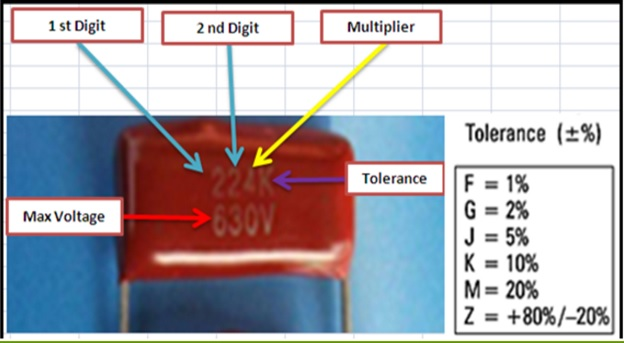## Saturday, May 7, 2016

### Understanding markings on capacitor body

For electrolytic capacitors the marking are easy to understand and many technician have no problem here.

The challenge is with non-polar capacitor, let have a look at some of the marking on non-polar capacitors.
Let have look at a few examples:Using the above method the result you get is always in Pico farad(pF)Let us look at the above example of capacitor number 224K.

Just write down the first and the second digit the way they are on the capacitor body.

So we have 22 and then third digit is the number of zeros. Therefore 4 is equal to 4 zeros hence 224 is equal to 220000 pF. The result is always in Pico farads (fF)

And the K is the tolerance and from the table we can see K is equal to (+ or -) 10 %, and finally the maximum voltage is 630 Volts.
Let us look at another example

So we have the first two digit 39 and add no zero, I mean you have 39 pF. If it was 391 then it could have been 39 then add one zero (0) and therefore could have been 39 0 pF again the tolerance is K therefore is +-10 %The maximum voltage is 1kV
We know 1K=1000 units, therefore (1kV=1000V)
Here is another example of non-polarized capacitor written on the body 1n0K

This is the same as 1.0n capacitor:
Given 1micro (uf) =1000nF
1nano=1000pf
So 1.0n is equal to 1000pF and a tolerance of +-10%
Maximum working voltage is 100Volts

Tip: encoding ceramic capacitor body markings:
White down the first two digits of the code, and then add the number of zeros that is indicated by the third digit of the code.
Examples:

Kindly note that capacitor 7n2=7k2=7200pF is one and the same thing.

B) 330 000pF
C) 22 000pF
D) 20 000 pF
If you did the quiz and got the answers right, then you should appreciate yourself with a cup of coffee.
That’s all for now friends

Humphrey Kimathi
Author Basic electronics course

Highly recommended eBooks by Jestine Yong

1.Thanks for the tutorial. Well explained and easy to understand.

1.Regards Humphrey

2.Thank you so much.

1.Welcome Joseph
Regards Humphrey

2.WONDERFUL AND EASY TO GRASP

3.your teaching is good my man

1.Thanks my Man
Regards Humphrey

4.I've been looking for a replacement for 1kV capacitor that looks like the orangey c) in the test above, but all that I can find are blue "disk" capacitors like example a). It seems all the 1KV 2KV and 3KV capacitors are labelled "High Voltage". I wonder if this is a new development in the capacitor world.